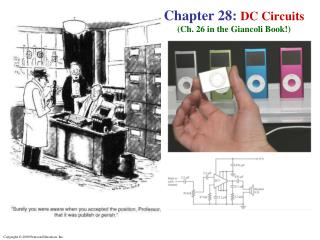Download PresentationChapter 28: DC Circuits (Ch. 26 in the Giancoli Book!)

# Chapter 28: DC Circuits (Ch. 26 in the Giancoli Book!) - PowerPoint PPT Presentation

Chapter 28: DC Circuits (Ch. 26 in the Giancoli Book!). EMF and Terminal Voltage. An electric circuit needs a battery or a generator to produce current – these are called Sources of “Electromotive Force” or EMF. It is important to remember that, despite its misleading name,I am the owner, or an agent authorized to act on behalf of the owner, of the copyrighted work described.
Download Presentation## Chapter 28: DC Circuits (Ch. 26 in the Giancoli Book!)

Download Policy: Content on the Website is provided to you AS IS for your information and personal use and may not be sold / licensed / shared on other websites without getting consent from its author.While downloading, if for some reason you are not able to download a presentation, the publisher may have deleted the file from their server.

- - - - - - - - - - - - - - - - - - - - - - - - - - E N D - - - - - - - - - - - - - - - - - - - - - - - - - -
Presentation Transcript
1. Chapter 28: DC Circuits(Ch. 26 in the Giancoli Book!)

2. EMF and Terminal Voltage An electric circuit needs a battery or a generator to produce current – these are called Sources of “Electromotive Force” or EMF. It is important to remember that, despite its misleading name, EMF is a VOLTAGE source! It isNOT a FORCE!! A battery is a nearly constant voltage source, but it does have a small internal resistance r, which reduces the actual voltage from the ideal EMF:

3. Example: Battery with Internal Resistance A resistor R = 65.0-Ω is connected to the terminals of a battery with emf E =12.0 V & internal resistance r = 0.5 Ω.Calculate: (a) the current I in the circuit, (b) the terminal voltage of the battery,Vab, and (c) the power dissipated in the resistor R and in the battery’s internal resistance r.

4. Resistors in Series & in Parallel A SERIES CONNECTION has a single path from the battery, through each circuit element in turn, then back to the battery.

5. The current through each resistor is the same; the voltage depends on the resistance. The sum of the voltage drops across the resistors equals the battery voltage:

6. This can be used to obtain the Equivalent Resistance Req(Req The single resistance that gives the same current in the circuit for the same voltage drop.) V  IReq

7. A PARALLEL CONNECTION splits the current; the voltage across each resistor is the same:

8. The total current I is the sum of currents across each resistor: , This gives the reciprocal of the equivalent resistance Req I  (V/Req)

9. An analogy using water may be helpful in visualizing parallel circuits. The water (current) splits into two streams; each falls the same height, and the total current is the sum of the two currents. With two pipes open, the resistance to water flow is half what it is with one pipe open.

10. Conceptual Example: Series or parallel? (a) The lightbulbs in the figure are identical. Which configuration produces more light? (b) Which way do you think the headlights of a car are wired? Ignore change of filament resistance R with current.

11. Conceptual Example: An illuminating surprise. A 100-W, 120-V lightbulb and a 60-W, 120-V lightbulb are connected in two different ways as shown.In each case, which bulb glows more brightly? Ignore change of filament resistance with current (and temperature).

12. Example: Circuit with series and parallel resistors. Calculate the current that is drawn from the battery shown.

13. Example: Current in one branch. Calculate the current I1 through the 500-Ω resistor.This is the same circuit as in the previous problem.)The total current I in the circuit was found to be I = 17 mA.

14. Conceptual Example: Bulb brightness in a circuit. The circuit shown has 3 identical lightbulbs, each of resistance R. (a) When switch S is closed, how will the brightness of bulbs A & B compare with that of bulb C? (b) What happens when switch S is opened? Use a minimum of mathematics in your answers.

15. Example: A two-speed fan. One way a multiple-speed ventilation fan for a car can be designed is to put resistors in series with the fan motor. The resistors reduce the current through the motor and make it run more slowly. Suppose the current in the motor is 5.0 A when it is connected directly across a 12-V battery. (a) What series resistor should be used to reduce the current to 2.0 A for low-speed operation? (b) What power rating should the resistor have?

16. Example: Analyzing a circuit. A 9.0-V battery with internal resistance r = 0.50 Ω is connected in the circuit shown. (a) How much current is drawn from the battery? (b) What is the terminal voltage of the battery? (c) What is the current in the 6.0-Ω resistor?